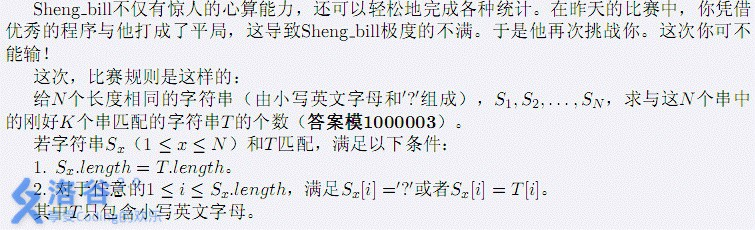# luogu 2167 [SDOI2009]Bill的挑战

### 题目描述T行整数，表示结果

1
2 1
a?
?b

50

### 题解

#include <cstdio>
#include <cstring>
using namespace std;
char s;
int f[1<<15];
int n,k,len,tot,mod=1000003;
int match;
void check(int x,int y)
{
for (int i=0;i<=len;i++)
if (!(s[x][i]=='?'||s[y][i]=='?'||s[x][i]==s[y][i]))
return;
match[x][y]=match[y][x]=1;
}
main()
{
int T;
scanf("%d",&T);
while(T--)
{
memset(f,0,sizeof f);
memset(match,0,sizeof match);
tot=0;
scanf("%d%d",&n,&k);
for (int i=1;i<=n;i++)
scanf("%s",s[i]);
len=strlen(s);

for (int i=0;i<len;i++)
for (char ch='a';ch<='z';ch++)
for (int j=1;j<=n;j++)
if (s[j][i]=='?'||s[j][i]==ch)
match[i][ch-'a']|=(1<<j-1);
int cnt=(1<<n)-1;
f[cnt]=1;
for (int i=0;i<len;i++)
for (int j=0;j<=cnt;j++)
if (f[i][j])
for (char ch='a';ch<='z';ch++)
f[i+1][match[i][ch-'a']&j]+=f[i][j],
f[i+1][match[i][ch-'a']&j]%=mod;
for (int i=0;i<=cnt;i++)
{
int ans=0;
for (int j=1;j<=cnt;j<<=1) ans+=(bool)(i&j);
if (ans==k) tot=(tot+f[len][i])%mod;
}
printf("%d\n",tot);
}
}